Pages - Self-Assessment for Grade 12 College Technology Math (MCT4C)
 Home About Us      Board of Trustees 2018 - 2022 Board of Trustees Roles and Responsibilities Multi-Year Plan Board and Committee Meetings Public Participation Policies, Procedures & By-Laws Integrity Commissioner Schools        Programs & Services Students Parents Community
 You Are Here :

## Quick Launch

Self-Assessment for Grade 12 College Technology Math (MCT4C)

Students who are registered for Grade 12 College Technology Math (MCT4C) may benefit from a self evaluation and review of the following sample of expectations from Grade 11 University/College Math (MCF3M).

The questions in this self-assessment reflect some of the key ideas learned in prerequisite courses.  They do not represent the problem solving approach or the rich experience that students would be exposed to in a classroom.  The intention is for students to revisit some key concepts and, if needed, access review materials in an informal environment at a pace that is comfortable for the student.

Concept

How comfortable do you feel with this concept?

1. Solve by factoring

a)b)c)I can determine the real roots of a variety of quadratic equations (i.e., graphing; factoring; using the quadratic formula)

2. Determine the roots of each quadratic.

a)b)I can sketch graphs ofby applying one or more transformations to the graph of3. For the quadratic relationa) Graph the Relation

State the:

b) direction of the opening;

c) coordinates of the vertex;

d) equation of the axis of symmetry;

e) y-intercept;

f) x-intercepts.Transformations of Parabolas

I can sketch graphs of quadratic functions in the factored formby using the x-intercepts to determine the vertex

4. Sketch the functionGraphing and Equations in Factored Form

I can solve problems arising from real-world applications, given the algebraic representation of a quadratic function.

5. Given the equation of a quadratic function representing the height of a ball over elapsed timea) Determine the maximum height of the ball.

b) Determine the length of time needed for the ball to touch the ground.

c) Determine the time interval when the ball is higher than 40m.I can evaluate, with and without technology, numerical expressions containing integer and rational exponents and rational bases

6. Evaluate

a)b)c)Exponent Laws all Together

I can describe key properties for exponential functions ( domain and range, intercepts, increasing/decreasing intervals, and asymptotes)

7. Describe key properties forProperties of Basic Exponential Functions

I can graph, with and without technology, an exponential relation, given its equation in the form8. Graph the functionTransformations of Exponential Functions

I can solve problems using given graphs or equations of exponential functions arising from a variety of real-world applications by interpreting the graphs

9. New Zealand has a population of about 6 million and it is estimated that the population will double in 36 years, modelled byIf population growth remains the same, what will the population be in 15 years? 40 years?Modelling with Exponential Functions

I can solve real-world application problems by using the primary trigonometric ratios, by determining the measures of the sides and angles of right triangles

10. To measure the height of a tall tree, a forester paces 40 m from the base of the tree and measures an angle of elevation to the top of the tree.  If the angle is, determine the height of the cedar tree.Sine and Cosine Ratios

I can solve problems that require the use of the sine law or the cosine law in acute triangles.

11. Two ships left Port Huron on Lake Ontario at the same time.  One travelled at 12 km/h on a course of.  The other travelled at 15km/h on a course of. How far apart are they four  hours later?The Sine Law

I can sketch graphs ofby applying the transformation to the graph ofand determine and describe its key properties (i.e., cycle, domain, range, intercepts, amplitude, period, maximum and minimum values, increasing/ decreasing intervals)

12. Considera) Sketch the graph over the interval.

b) Describe its key properties.Investigate Transformations of Sinusoidal Functions

I can identify periodic and sinusoidal functions

13. Which of the following scenarios could be modelled by periodic functions?

a) Sunset times in Aurora, Ontario.

b) The motion of a planet around the sun.

c) A child’s movement on a swing.

d) The growth of a bacteria.Modelling Periodic Behaviour

## Solutions to Sample Questions

1. Solve by factoring

a)b)c)Solutions:

a)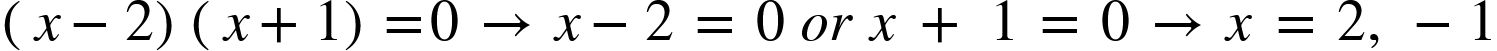b)

c)2. Determine the roots of each quadratic.

a)b)Solutions:

a)b)3. For the quadratic relationa) Graph the Relation

State the:

b) direction of the opening;

c) coordinates of the vertex;

d) equation of the axis of symmetry;

e) y-intercept;

f) x-intercepts.

Solutions:

1.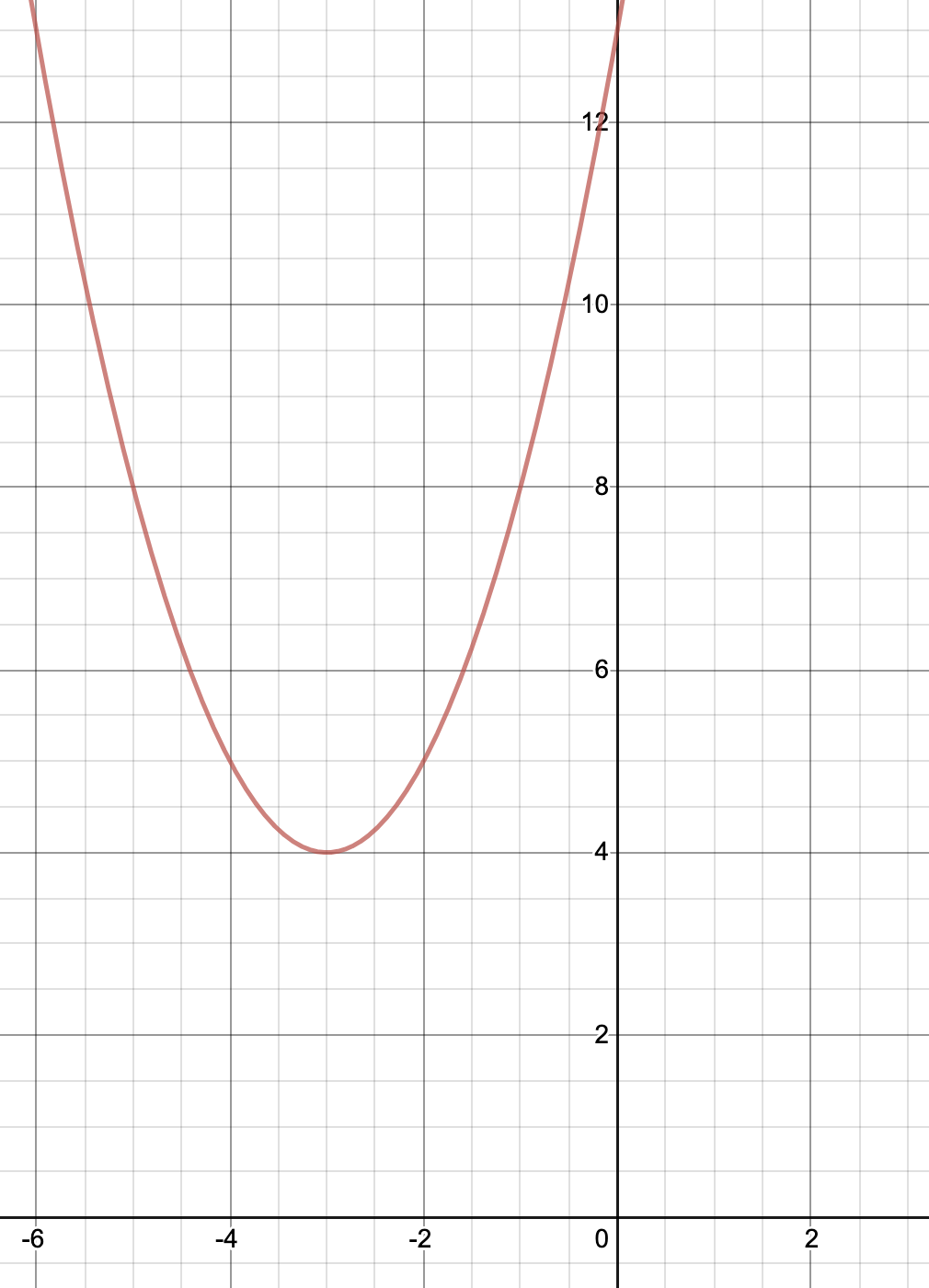b)  up

c)d)e)f) there are no x-intercepts for this function.

4. Sketch the functionSolution:

Use the x-intercepts to plot.  Sketch the axis of symmetry as. Locate the vertex using substitution of.  Connect intercepts and vertex to sketch.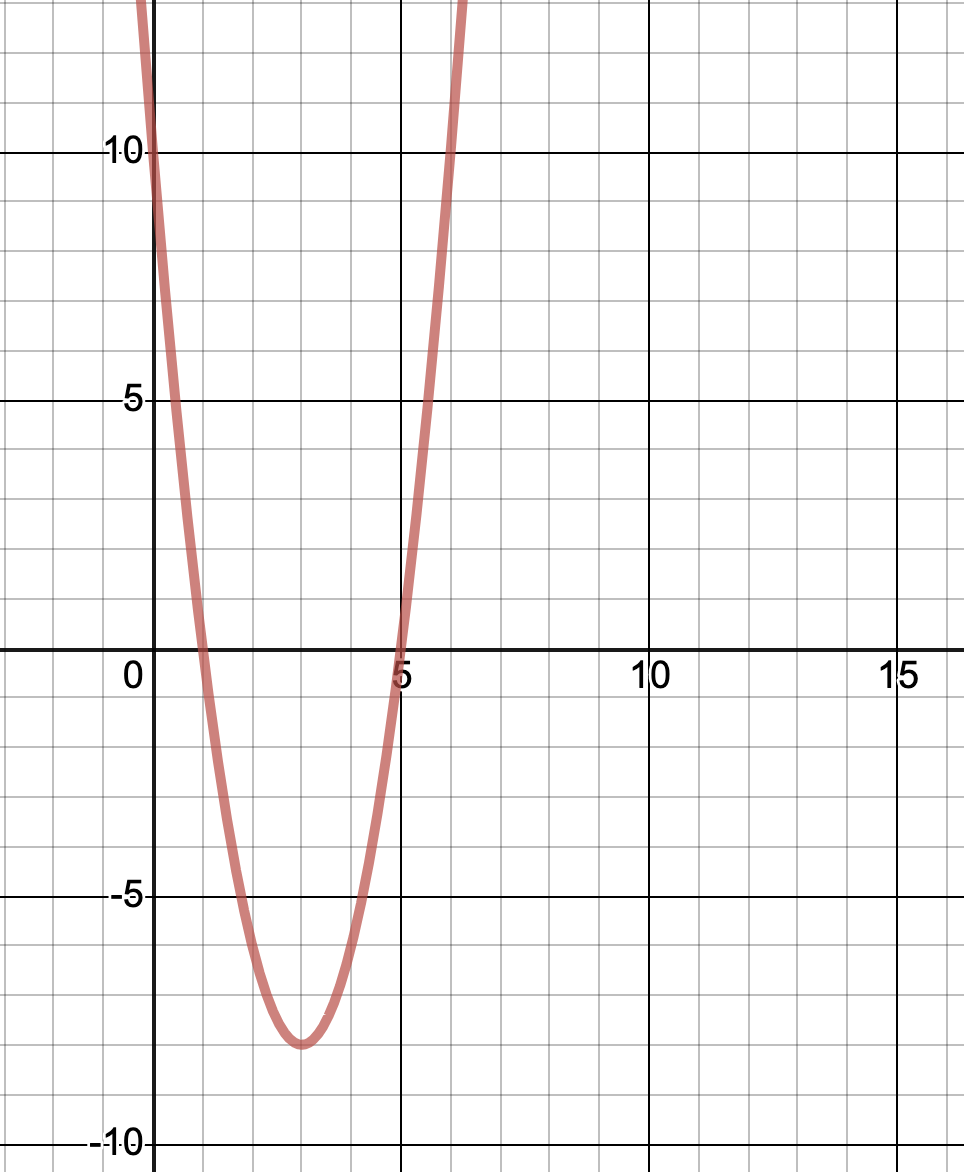5. Given the equation of a quadratic function representing the height of a ball over elapsed timea) Determine the maximum height of the ball.

b) Determine the length of time needed for the ball to touch the ground.

c) Determine the time interval when the ball is higher than 40m.

Solutions: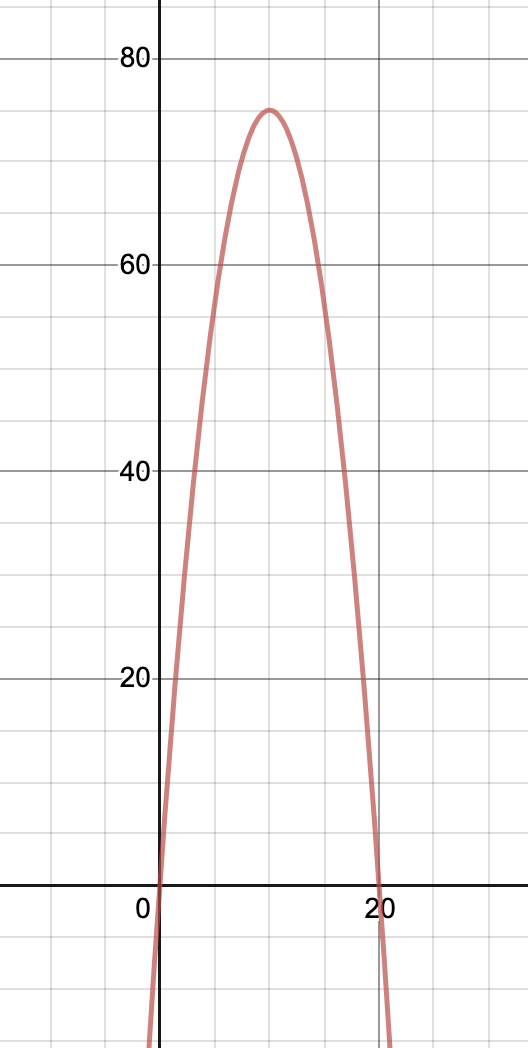a) Max height 75m

b) 20 seconds

c)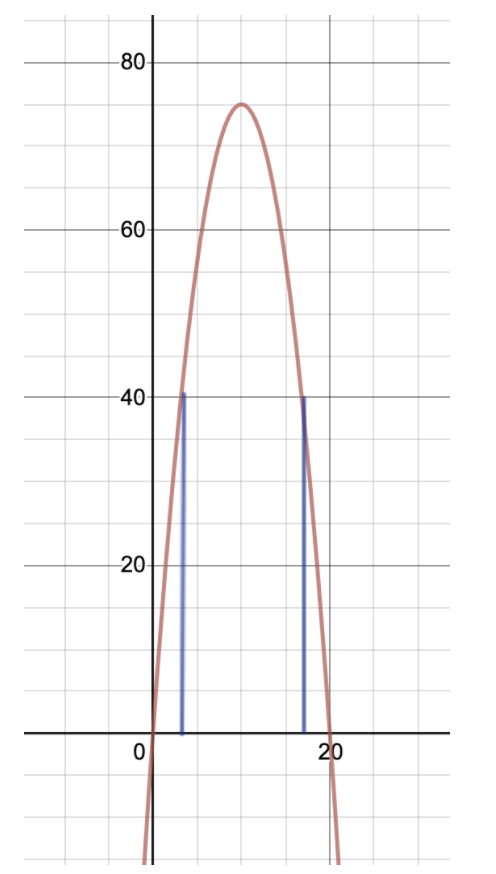Using  interpolation - it appears that the height is greater than 40m from approximately 3 seconds to approximately 17 seconds, for a total of approximately 14 seconds.

Alternatively, one could also solve the equationto get 13.7 seconds.

6. Evaluate

a)b)c)Solutions:a)

b)c)or7. Describe key properties forSolution:

decay curve.

decreasing function .

Rate of decay =.

y-intercept at 200.8. Graph the function

Solution:9. New Zealand has a population of about 6 million and it is estimated that the population will double in 36 years, modelled by.  If population growth remains the same, what will the population be in 15 years? 40 years?

Solutions:

a)million

b)million

10. To measure the height of a tall tree, a forester paces 40 m from the base of the tree and measures an angle of elevation to the top of the tree.  If the angle is, determine the height of the cedar tree.

Solution: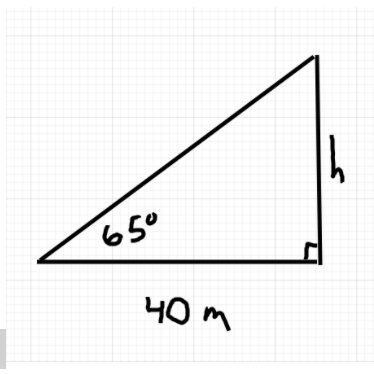Therefore the height of the tree is 86m.

11. Two ships left Port Huron on Lake Ontario at the same time.  One travelled at 12 km/h on a course of.  The other travelled at 15km/h on a course of. How far apart are they four hours later?

Solution: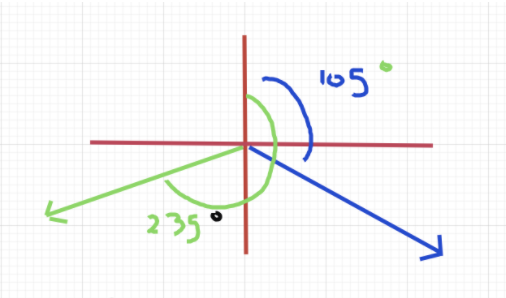From the diagram, the obtuse angle formed between the directions of the two ships is.

After four hours of travel, the ships have travelled respectively 48 km and 60km.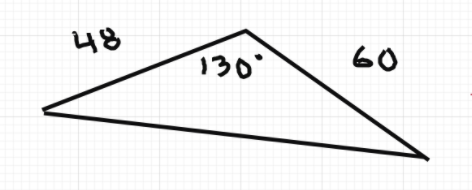Therefore the two ships are 98km apart after 4 hours.

12. Considera) Sketch the graph over the interval.

b) Describe its key properties.

Solutions:

a)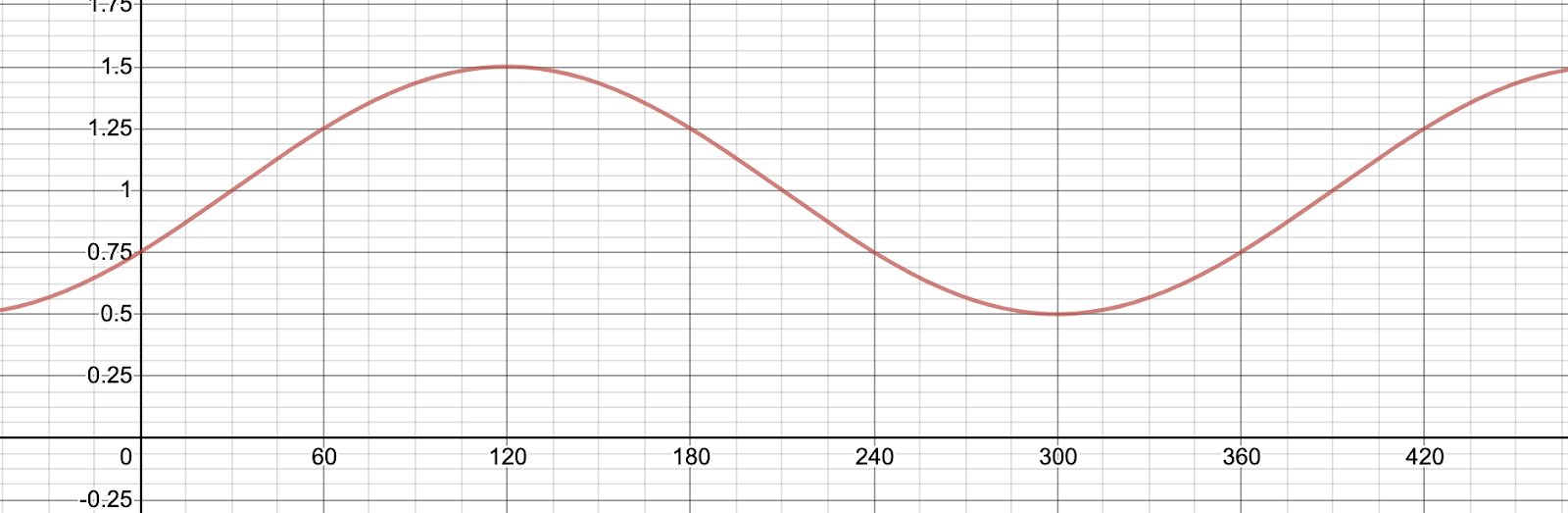b) Amplitude = 0.5.  Period length =. Phase shift ofright. Vertical translation of 1 unit up. Maximum at 1.5.  Minimum at 0.5.

13. Which of the following scenarios could be modelled by periodic functions?

a) Sunset times in Aurora, Ontario

b) The motion of a planet around the sun

c) A child’s movement on a swing

d) The growth of a bacteria

Solutions:

a and b only.